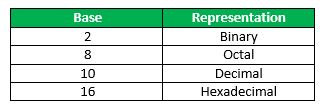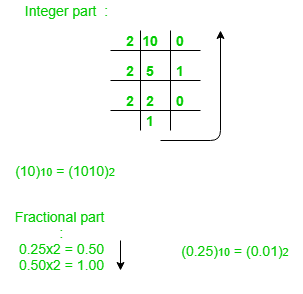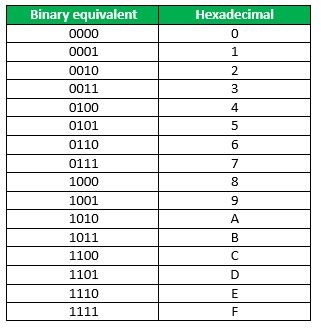GFG App
Open AppBrowser
Continue

# Number System and Base Conversions

Electronic and Digital systems may use a variety of different number systems, (e.g. Decimal, Hexadecimal, Octal, Binary), or even Duodecimal or less well known but better named Uncial. All the other bases other than Decimal result from computer usage. Uncial (named from Latin for 1/12 “uncia” the base twelve analogue of Decimal from the Latin word for 1/10 “decima”).

A number N in base or radix b can be written as:

`(N)b = dn-1 dn-2 -- -- -- -- d1 d0 . d-1 d-2 -- -- -- -- d-m`

In the above, dn-1 to d0 is the integer part, then follows a radix point, and then d-1 to d-m is the fractional part.

dn-1 = Most significant bit (MSB)
d-m = Least significant bit (LSB)How to convert a number from one base to another?

## 1. Decimal to Binary

(10.25)10Note: Keep multiplying the fractional part with 2 until decimal part 0.00 is obtained.
(0.25)10 = (0.01)2

## 2. Binary to Decimal

```(1010.01)2
1x23 + 0x22 + 1x21+ 0x20 + 0x2 -1 + 1x2 -2 = 8+0+2+0+0+0.25 = 10.25
(1010.01)2 = (10.25)10 ```

## 3. Decimal to Octal

```(10.25)10
(10)10 = (12)8
Fractional part:
0.25 x 8 = 2.00 ```

Note: Keep multiplying the fractional part with 8 until decimal part .00 is obtained.
(.25)10 = (.2)8

## 4. Octal to Decimal

```(12.2)8
1 x 81 + 2 x 80 +2 x 8-1 = 8+2+0.25 = 10.25
(12.2)8 = (10.25)10 ```

To convert from Hexadecimal to Binary, write the 4-bit binary equivalent of hexadecimal.(3A)16 = (00111010)2

To convert from Binary to Hexadecimal, start grouping the bits in groups of 4 from the right-end and write the equivalent hexadecimal for the 4-bit binary. Add extra 0’s on the left to adjust the groups.

```1111011011
0011 1101 1011
(001111011011 )2 = (3DB)16 ```

## 7. Binary to Octal

To convert from binary to octal, start grouping the bits in groups of 3 from the right end and write the equivalent octal for the 3-bit

Example:

111101101

111 101 101

(111101101)2 = (755)8